Courses

# Plastic Analysis Civil Engineering (CE) Notes | EduRev

## Civil Engineering (CE) : Plastic Analysis Civil Engineering (CE) Notes | EduRev

The document Plastic Analysis Civil Engineering (CE) Notes | EduRev is a part of the Civil Engineering (CE) Course Topic wise GATE Past Year Papers for Civil Engineering.
All you need of Civil Engineering (CE) at this link: Civil Engineering (CE)

Q.1 If the section shown in figure turns from fully elastic to fully plastic, the depth of neutral axis (NA),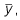decreases by    [2019 : 2 Marks, Set-I]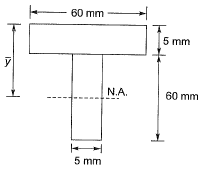(a) 12.25 mm
(b) 15.25 mm
(c) 10.75 mm
(d) 13.75 mm
Ans.
(D)
Solution: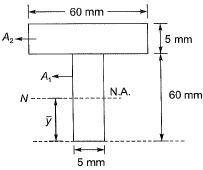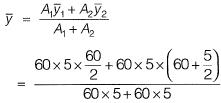= 46.25 mm
NA - Neutral axis
The section is unsymmetrical about the NA and hence the equal area axis (EA) has to be located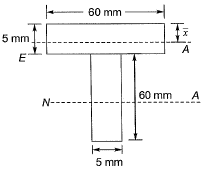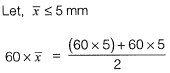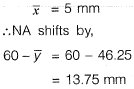Q.2  The dimension of a symmetrical welded l-section are shown in the figure.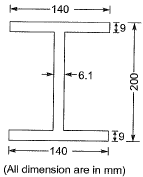The plastic section modulus about the weaker axis (in cm3, up to one decimal place) is _____.    [2018 : 2 Marks, Set-I]
Solution: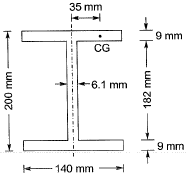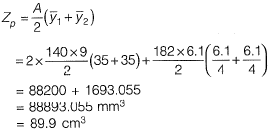Note: Keep in wind the units and decimal places.

Q.3 A fixed-end beam is subjected to a concentrated load (P) as shown in the figure. The beam has two different segments having different plastic moment capacities  (MP, 2Mp) as shown.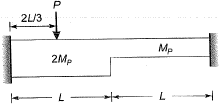The minimum value of load (P) at which the beam would collapse (ultimate load is)     [2016 : 2 Marks, Set-II]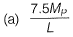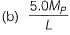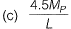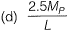Ans.
(A)
Solution:
Ds = 2
∴ Number of plastic hinge required for complete collapse = Ds + 1
= 2 + 1 = 3
Mechanism 1: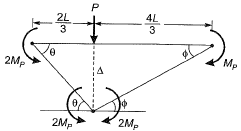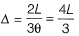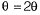For principal of virtual work done,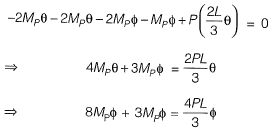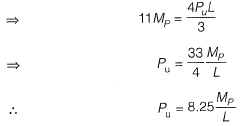Mechanism 2: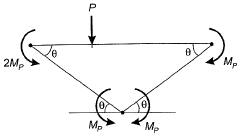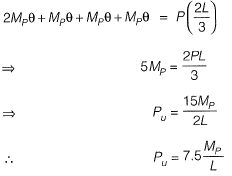Q.4   A fixed end beam is subjected to a load, W at 1 /3rd span from the left support as shown in the figure. The collapse load of the beam is    [2015 : 2 Marks, Set-II]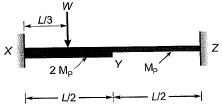(a) 16.5 Mp/L
(b) 15.5 Mp/L
(c) 15.0 Mp/L
(d) 16.0 Mp/L
Ans.
(C)
Solution:
No. of plastic hinges formed at collapse
= r + 1 = 3
There can be two collapse mechanisms
Case (I)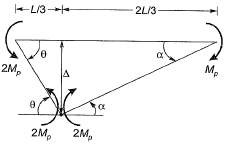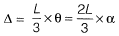⇒ θ = 2α
Internal work done = External work done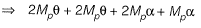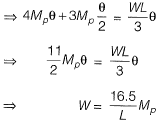Case (II)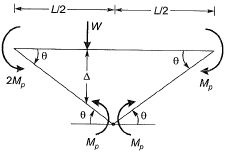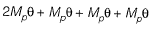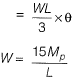Hence, minimum collapse load is 15Mp/L.

Q.5  For formation of collapse mechanism in the following figure, the minimum value of Pu is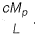Mp and 3Mp denote the plastic moment capacities of beam sections as shown in this figure. The value of c is ____.    [2015 : 2 Marks, Set-I]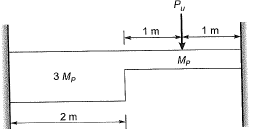Solution:
As L is not defined in question. So, by assuming L = 1 m
Mechanism 1: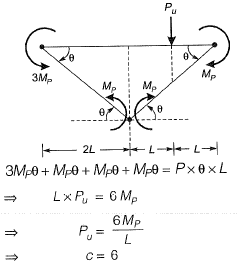Mechanism 2: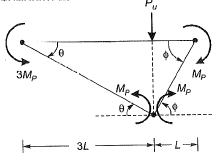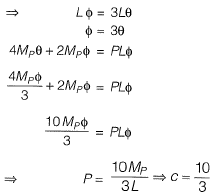Failure load obtained out of two mechanisms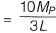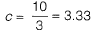Q.6 A prismatic beam (as shown below) has plastic moment capacity of M , then the collapse load MP of the beam is    [2014 : 2 Marks, Set-II]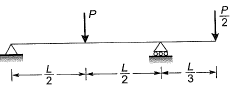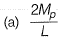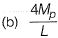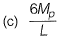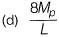Ans.
(C)
Solution:
Here degree of static indeterminacy = 0
∴ Number of plastic hinges required for mechanical equlibrium
= Ds + 1 = 0 + 1 = 1
Mechanism 1: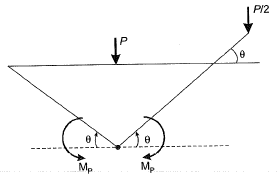From principal of virtual work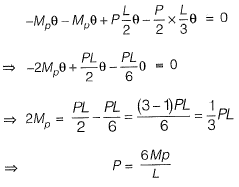Mechanism 2: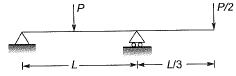From principal of virtual work, equating external work donw to internal work done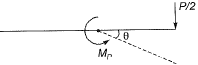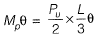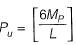Q.7  The ultimate collapse load (P) in terms of plastic moment Mp by kinematic approach for a propped cantilever of length L with Pacting at its mid-span as shown in the figure, would be    [2014 : 1 Mark, Set-I]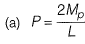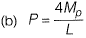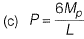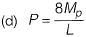Ans.
(C)
Solution: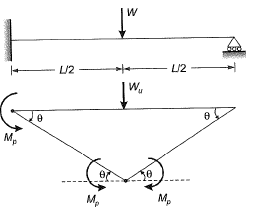From principal of virtual work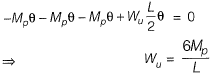Q.8   A propped cantilever made of a prismatic steel beam is subjected to a concentrated load P at mid span as shown.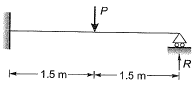If the magnitude of load Pis increased till collapse and the plastic moment carrying capacity of steel beam section is 90 kNm, determine reaction R (in kN) (correct to 1-decimal place) using plastic analysis ________.    [2013 : 2 Marks]
Solution: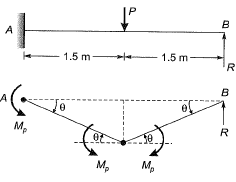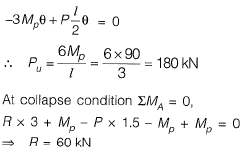Q.9  A propped cantilever made of a prismatic steel beam is subjected to a concentrated load P at mid span as shown.If load P = 80 kN, find the reaction R (in kN) (correct to 1 decimal place) using elastic analysis______.    [2013 : 2 Marks]
Solution:

Equating deflection at end B,
Let, l = 3 m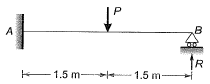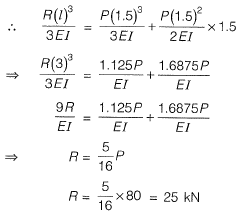Q.10  As per IS 800 : 2007 the cross-section in which extreme fibre can reach the yield stress but can not develop the plastic moment of resistance due to local buckling is classified as    [2013 : 1 Mark]
(a) plastic section
(b) compact section
(c) semi compact section
(d) shear section
Ans.
(C)
Solution:
Plastic section: Cross-section which can develop plastic hinges and have the rotation capacity required for the failure of the structure by formation of plastic mechanism.
Compact section: Cross-section which can develop plastic moment of resistance but have inadequate plastic hinge rotation capacity for formation of plastic mechanism before buckling.
Semi compact: Cross-sections, in which the expense fibre in compression can reach yield stress, but cannot develop plastic moment of resistance due to location buckling.
Slender: Cross-sections in which the elements buckle locally even before attainment of yield stress are closed as sledner sections.

Offer running on EduRev: Apply code STAYHOME200 to get INR 200 off on our premium plan EduRev Infinity!

69 docs

,

,

,

,

,

,

,

,

,

,

,

,

,

,

,

,

,

,

,

,

,

;